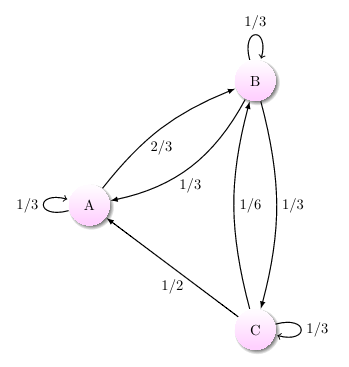Probability

# Markov Chains - Transition MatricesSuppose a process begins at one state and progresses to another state after 3 turns. Which pair $($start, end$)$ is most likely?

A Markov chain has transition matrix $\begin{pmatrix} 0.5 & 0.3 & 0.2 \\ 0.4 & 0.4 & 0.2 \\ 0.6 & 0.4 & 0 \end{pmatrix}.$

What is its $2$-step transition matrix?

(A): $\begin{pmatrix} 0.49 & 0.35 & 0.16 \\ 0.48 & 0.36 & 0.16 \\ 0.46 & 0.34 & 0.2 \end{pmatrix}$

(B): $\begin{pmatrix} 0.25 & 0.35 & 0.4 \\ 0.39 & 0.25 & 0.36 \\ 0.36 & 0.39 & 0.25 \end{pmatrix}$

(C): $\begin{pmatrix} 0.49 & 0.35 & 0.16 \\ 0.48 & 0.36 & 0.16 \\ 0.48 & 0.32 & 0.2 \end{pmatrix}$

(D): $\begin{pmatrix} 0.49 & 0.35 & 0.16 \\ 0.4 & 0.44 & 0.16 \\ 0.48 & 0.32 & 0.2 \end{pmatrix}$

Phineas is flipping a fair coin repeatedly and marking down the results. However, he has a soft spot for heads, so half the time he marks heads, he marks heads again. (This means he could possibly mark down heads many times in a row without actually flipping the coin again.) The resulting sequence of states could be modeled by a Markov chain, where the first possible state (and row of the matrix) is heads and the second possible state is tails. What is its transition matrix?

(A): $\begin{pmatrix} \tfrac{1}{2} & \tfrac{1}{2} \\ \tfrac{1}{2} & \tfrac{1}{2} \end{pmatrix}$

(B): $\begin{pmatrix} \tfrac{1}{2} & \tfrac{1}{2} \\ \tfrac{1}{4} & \tfrac{3}{4} \end{pmatrix}$

(C): $\begin{pmatrix} \tfrac{3}{4} & \tfrac{1}{2} \\ \tfrac{1}{4} & \tfrac{1}{2} \end{pmatrix}$

(D): $\begin{pmatrix} \tfrac{3}{4} & \tfrac{1}{4} \\ \tfrac{1}{2} & \tfrac{1}{2} \end{pmatrix}$

A Markov chain can be constructed for the weather based on the following table.

 States Rainy Tomorrow Cloudy Tomorrow Sunny Tomorrow Rainy Today $\tfrac{1}{2}$ $\tfrac{1}{3}$ $\tfrac{1}{6}$ Cloudy Today $\tfrac{1}{3}$ $\tfrac{1}{3}$ $\tfrac{1}{3}$ Sunny Today $0$ $\tfrac{1}{9}$ $\tfrac{8}{9}$

If it is rainy today, what is the probability that it will be rainy in three days?

If a Markov chain $\{X_0, \, X_1, \, \dots\}$ has transition matrix $P$ and states $i$ and $j$, then what is the value of $P_{i,j}$?

×